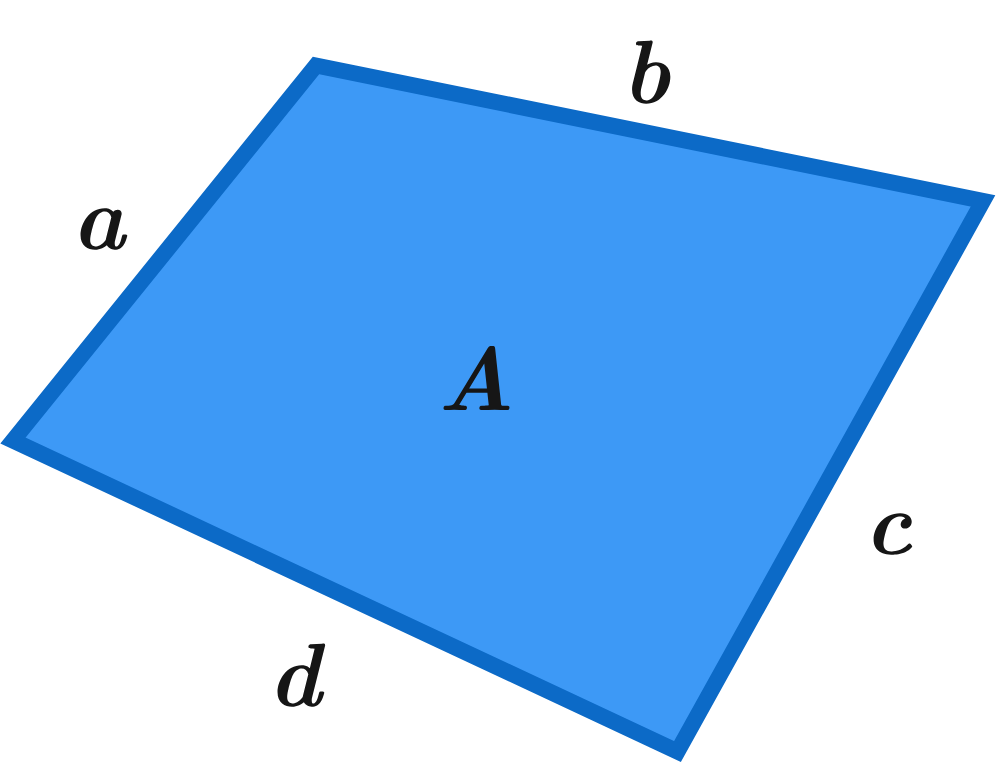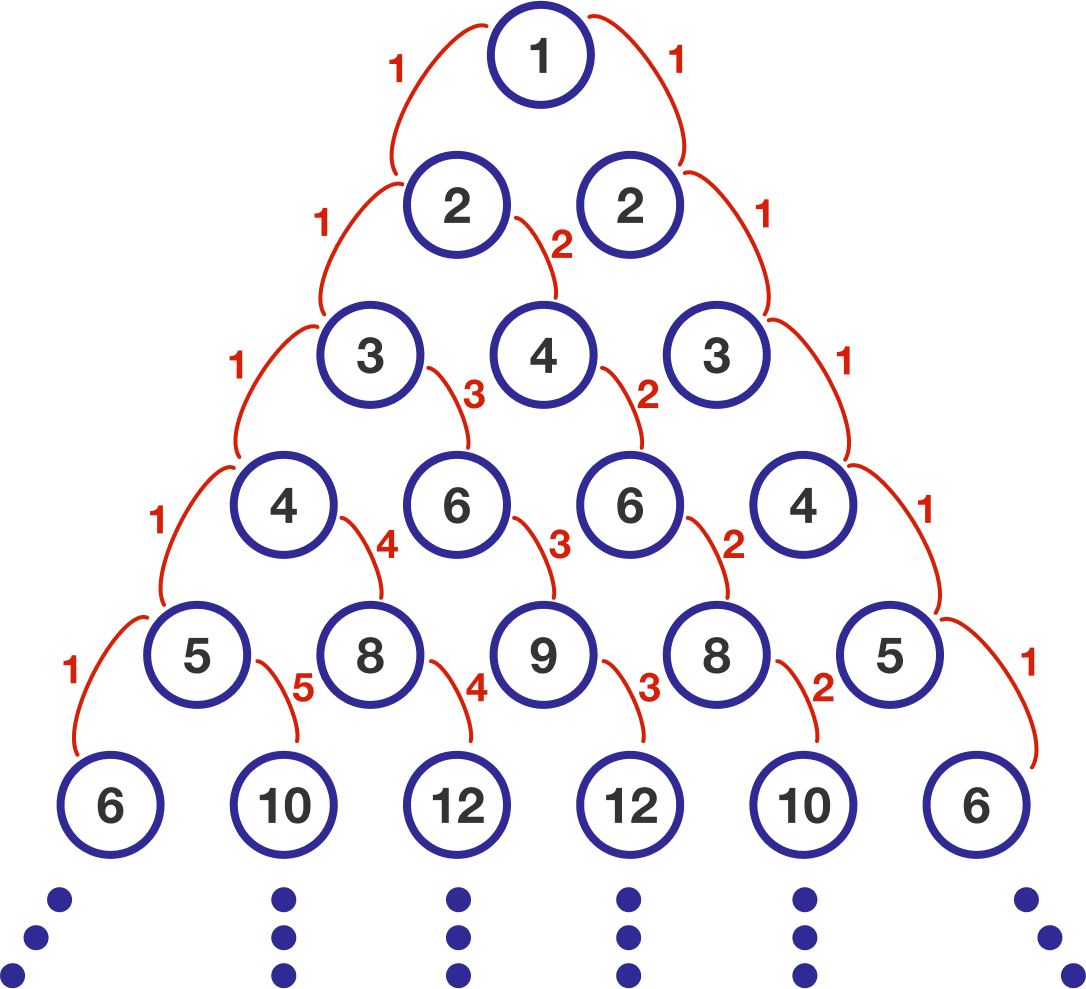# Problems of the Week

Contribute a problem

# 2018-09-17 Basic

Astronauts in microgravity aboard the International Space Station can't use a normal scale to weigh themselves. One way they measure their mass is by riding a large compressed spring.

When the spring is released, a computer compares the motion to how the spring moves without the added mass of the person.

What will happen to the motion of the spring when the astronaut gets on the spring?

If we know all 4 side lengths of a quadrilateral, can we determine the area of the quadrilateral?Note: Heron's formula allows one to determine the area of a triangle given the 3 side lengths.

True or False?

If you add a positive number to its reciprocal, then the result is always greater than or equal to 2.

$x + \frac{1}{x} \ge 2$

Bonus: If true, give the relevant proof; if false, explain why.

A clock with no numbers fell to the ground.

What time is it?The numbers in the circles follow the pattern shown below.In which horizontal row of circles does the number $2018$ first appear?

×